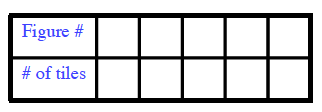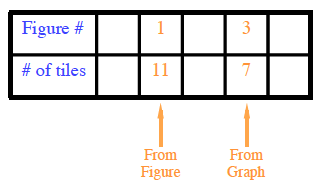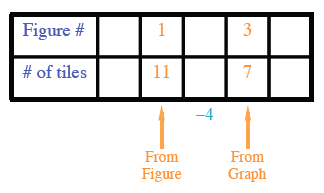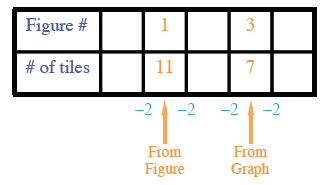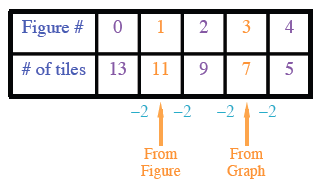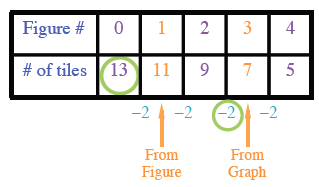### Home > CCA > Chapter 3 > Lesson 3.2.4 > Problem3-71

3-71.

Find the rule for the pattern represented at right.

 tile tile tile tile tile tile tile tile tile tile Tile

Figure 1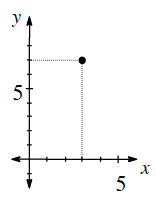Create a table to help find a pattern.A table with 2 rows and 6 columns. Entered in the left column are Figure number and number of tiles.

Fill in the information that you are given. This table is a continuation of the table above with 1 in the top 3rd column, 3 in the top 5th column, 11 in the bottom 3rd column, and 7 in the bottom 5th column. The numbers inputted in the 3rd column are from the figure and the numbers inputted in the 5th column are from the graph.

What is the difference between the number of tiles in the two figures? This table is a continuation of the table above. Negative 4 is written below the fourth column.

Use this information to find the difference between the number of tiles in consecutive figures.  This table is a continuation of the table above. Minus 2 is written below the dividing line between adjacent columns from column 2 to column 6.

Now fill in the rest of the table.
This table is a continuation of the table above with 0 in the top 2nd cell, 2 in the top 4th cell, 4 in the top 6th cell, 13 in the bottom 2nd cell, 9 in the bottom 4th cell, and 5 in the bottom 6th cell.

This table is a continuation of the table above with a Circle around the 13 in the bottom row and a circle around the minus 2 between columns 4 and 5.Using the starting point and the growth rate, write an equation to represent the pattern.